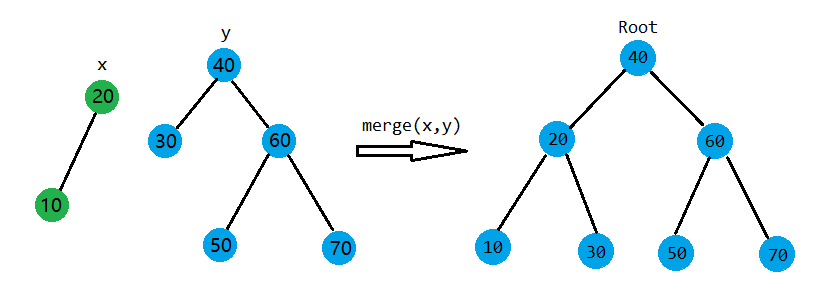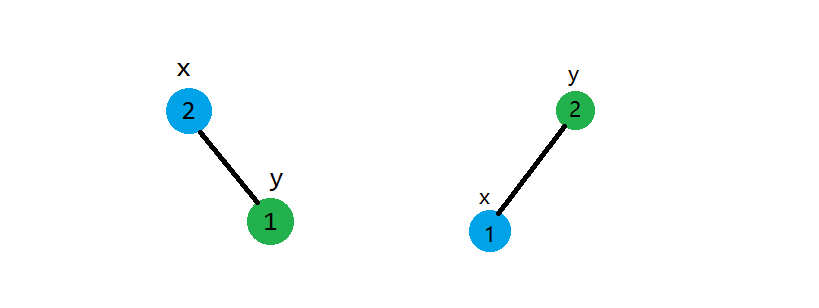2020/01/26 20:33

# FHQ Treap 是什么？

<div id="copyright"> Copyright © 2019 ctjcalc，转载请注明URL，并给出原文链接，谢谢。 </div> FHQ Treap，又名无旋Treap，是一种不需要旋转的平衡树，是**范浩强**基于Treap发明的。FHQ Treap具有代码短，易理解，速度快的优点。（当然跟红黑树等更高级的平衡树比一下就是……）至少它在OI中算是很优秀的数据结构了。

• C++
• 二叉搜索树的基本性质，下面会讲
• 二叉堆

# 二叉搜索树的基本性质

• 二叉搜索树中，每个结点都满足左子树的结点的值都小于等于自己的值，右子树的结点的值都大于自己的值，左右子树也是二叉搜索树。
• 中序遍历二叉搜索树可以得到一个由这棵树的所有结点的值组成的有序序列。（即所有的值排序后的结果）

# 原理&代码实现

FHQ Treap不是通过旋转来保持平衡的，而是通过两个函数splitmerge。顾名思义，split就是分裂，merge就是合并。当然，从最底层的原理来看，还不是这两个函数。FHQ Treap中的Treap代表Tree + Heap，也就是说，FHQ Treap会按二叉搜索树一样根据键值排序结点，并且随机赋给每个结点一个优先级，按照二叉堆的顺序排序结点（这里用大根堆）。Treap通过旋转，使平衡树同时满足这两个性质，从而达到平衡。而FHQ Treap通过调用merge函数时使平衡树满足堆序，实现原理与Treap不同。

## 结点信息

<div id="copyright"> Copyright © 2019 ctjcalc，转载请注明URL，并给出原文链接，谢谢。 </div> FHQ Treap是一个二叉树，所以可以写出这样的代码：

template <typename T, int MaxSize>
class FHQTreap
{
public:
FHQTreap() { Seed = (int)(MaxSize * 565463ll % 2147483647); }
// ...
private:
struct Node
{
T Key;
int Left, Right, Size, Priority;
} Tree[MaxSize];
int Seed, Total, Root;

int random() { return Seed = (int)(Seed * 104831ll % 0x7fffffff); }

void pushup(int root) {
if (root != 0) {
Tree[root].Size = Tree[Tree[root].Left].Size + Tree[Tree[root].Right].Size + 1; // + 1是要算上自己
}
}
// ...
}


Node即结点，里面的Key就是要存的值，Priority即优先级。Seed就是随机数种子，在构造函数中初始化，random()会生成一个在int范围内的整数，作为结点的优先级。（我自己写随机数生成函数只是个人习惯）

## 构造新结点

int create(T key) {
int root = ++Total;
Tree[root].Key = key;
Tree[root].Size = 1;
Tree[root].Left = Tree[root].Right = 0;
return root;
}


create(T key)会初始化一个结点，并返回它的ID，大家也可以用指针实现。这里比较简单，就不多解释了。

## split函数

split分为两种：

• 按值分裂：根据一个值$key$把一棵树分裂成两棵树，一棵树的值全部小于等于$key$，另外一棵全部大于$key$
• 按大小分裂：根据一个值$size$分裂树，一棵的大小为$size$，另外一棵为剩下的。

### 按值分裂

void split(int root, int key, int &x, int &y) { // x, y即分裂出的两个树
if (root == 0) {
x = y = 0;
return;
}
if (!(key < Tree[root].Key)) { // 等价于 Tree[root].Key <= key
x = root; // 把root设为x树的根（当前）
split(Tree[root].Right, key, Tree[root].Right, y); // 找更大的结点
} else {
y = root; // 相反过程
split(Tree[root].Left, key, x, Tree[root].Left);
}
pushup(root); // 记得更新大小
}


### 按大小分裂

<div id="copyright"> Copyright © 2019 ctjcalc，转载请注明URL，并给出原文链接，谢谢。 </div> 与按值分裂类似，把值换成大小，注意递归右子树时要把$size$减去$Size_{Left_{x}}+1$，这也是显然的。

void split(int root, int sze, int &x, int &y) {
if (root == 0) {
x = y = 0;
return;
}
if (Tree[Tree[root].Left].Size + 1 <= sze) {
x = root;
split(Tree[root].Right, sze - Tree[Tree[root].Left].sze - 1, Tree[root].Right, y);
} else {
y = root;
split(Tree[root].Left, sze, x, Tree[root].Left);
}
pushup(root);
}


## merge函数int merge(int x, int y) {
if (x == 0 || y == 0)
return x + y;
/*
如果其中一个结点为空，即只剩另下一棵树需要处理，就直接返回
因为空结点的ID为0，所以直接返回 x + y 即可。 如果两棵树都为空，这样也是没有问题的。
*/
if (Tree[x].Priority > Tree[y].Priority) {
Tree[x].Right = merge(Tree[x].Right, y);
pushup(x);
return x;
} else {
Tree[y].Left = merge(x, Tree[y].Left);
pushup(y);
return y;
}
}


## 各种修改&查询

void insert(T key) {
int x, y;
split(Root, key - 1, x, y);
Root = merge(merge(x, create(key)), y);
}


### 删除

void remove(T key) {
int x, y, z;
split(Root, key, x, z);
split(x, key - 1, x, y);
if (y) { // 如果删除所有，就直接去掉这个if语句块，并且下面的只合并x, z
y = merge(Tree[y].Left, Tree[y].Right);
}
Root = merge(merge(x, y), z);
}


### 查询指定值的排名

FHQ Treap上，我们就直接按$key-1$分裂树，查一下值小于等于$key-1$的树的大小，再$+1$即可。

int rank(T key) {
int x, y, ans;
split(Root, key - 1, x, y);
ans = Tree[x].Size + 1;
Root = merge(x, y);
return ans;
}


### 查询指定排名的值

#### 写法1

• $size+1=rank$，找到答案
• $size+1>rank$，在左子树
• $size+1<rank$，在右子树
T at(int r) {
int root = Root;
while (true) {
if (Tree[Tree[root].Left].Size + 1 == r) {
break;
} else if (Tree[Tree[root].Left].Size + 1 > r) {
root = Tree[root].Left;
} else {
r -= Tree[Tree[root].Left].Size + 1;
root = Tree[root].Right;
}
}
return Tree[root].Key;
}


#### 写法2

// 这里的split是按大小分裂
T at(int r) {
int x, y, z;
split(Root, r - 1, x, y);
split(y, 1, y, z);
T ans = Tree[y].Key;
Root = merge(merge(x, y), z);
return ans;
}


### 查询前驱

T prev(T key) {
int x, y, root;
T ans;
split(Root, key - 1, x, y);
root = x;
while (Tree[root].Right) root = Tree[root].Right;
ans = Tree[root].Key;
Root = merge(x, y);
return ans;
}


### 查询后继

T next(T key) {
int x, y, root;
T ans;
split(Root, key, x, y);
root = y;
while (Tree[root].Left) root = Tree[root].Left;
ans = Tree[root].Key;
Root = merge(x, y);
return ans;
}


### 查询树的大小

int size() {
return Tree[Root].Size;
}


### 查询一个元素是否存在

bool find(T key) {
int x, y, z;
split(Root, key, x, z);
split(x, key - 1, x, y);
bool ans;
if (Tree[y].Size) ans = true;
else ans = false;
Root = merge(merge(x, y), z);
return ans;
}


## 垃圾回收优化

// Stack[]即栈，用来存储结点，也可以使用std::stack<T>
void remove(T key) {
int x, y, z;
split(Root, key, x, z);
split(x, key - 1, x, y);
if (y) {
if(Top < (MaxSize >> 8) - 5) Stack[++Top] = y;
y = merge(Tree[y].Left, Tree[y].Right);
}
Root = merge(merge(x, y), z);
}

int create(T key) {
int root = Top ? Stack[Top--] : ++Total;
Tree[root].Key = key;
Tree[root].Size = 1;
Tree[root].Left = Tree[root].Right = 0;
return root;
}


# 例题

## 文艺平衡树

void pushdown(int rt) {
if (tree[rt].rev == 0)
return;
swap(tree[rt].l, tree[rt].r);
tree[tree[rt].l].rev ^= 1;
tree[tree[rt].r].rev ^= 1;
tree[rt].rev = 0;
}

void split(int rt, int sze, int &x, int &y) {
if (rt == 0) {
x = y = 0;
return;
}
pushdown(rt);
if (tree[tree[rt].l].sze + 1 <= sze) {
x = rt;
split(tree[rt].r, sze - tree[tree[rt].l].sze - 1, tree[rt].r, y);
} else {
y = rt;
split(tree[rt].l, sze, x, tree[rt].l);
}
pushup(rt);
}

int merge(int x, int y) {
if (x == 0 || y == 0)
return x + y;
if (tree[x].pri > tree[y].pri) {
pushdown(x);
tree[x].r = merge(tree[x].r, y);
pushup(x);
return x;
} else {
pushdown(y);
tree[y].l = merge(x, tree[y].l);
pushup(y);
return y;
}
}

void reverse(int l, int r) {
int x, y, z;
split(root, l - 1, x, y);
split(y, r - l + 1, y, z);
tree[y].rev ^= 1;
root = merge(merge(x, y), z);
}


0
0 收藏

### 作者的其它热门文章0 评论
0 收藏
0